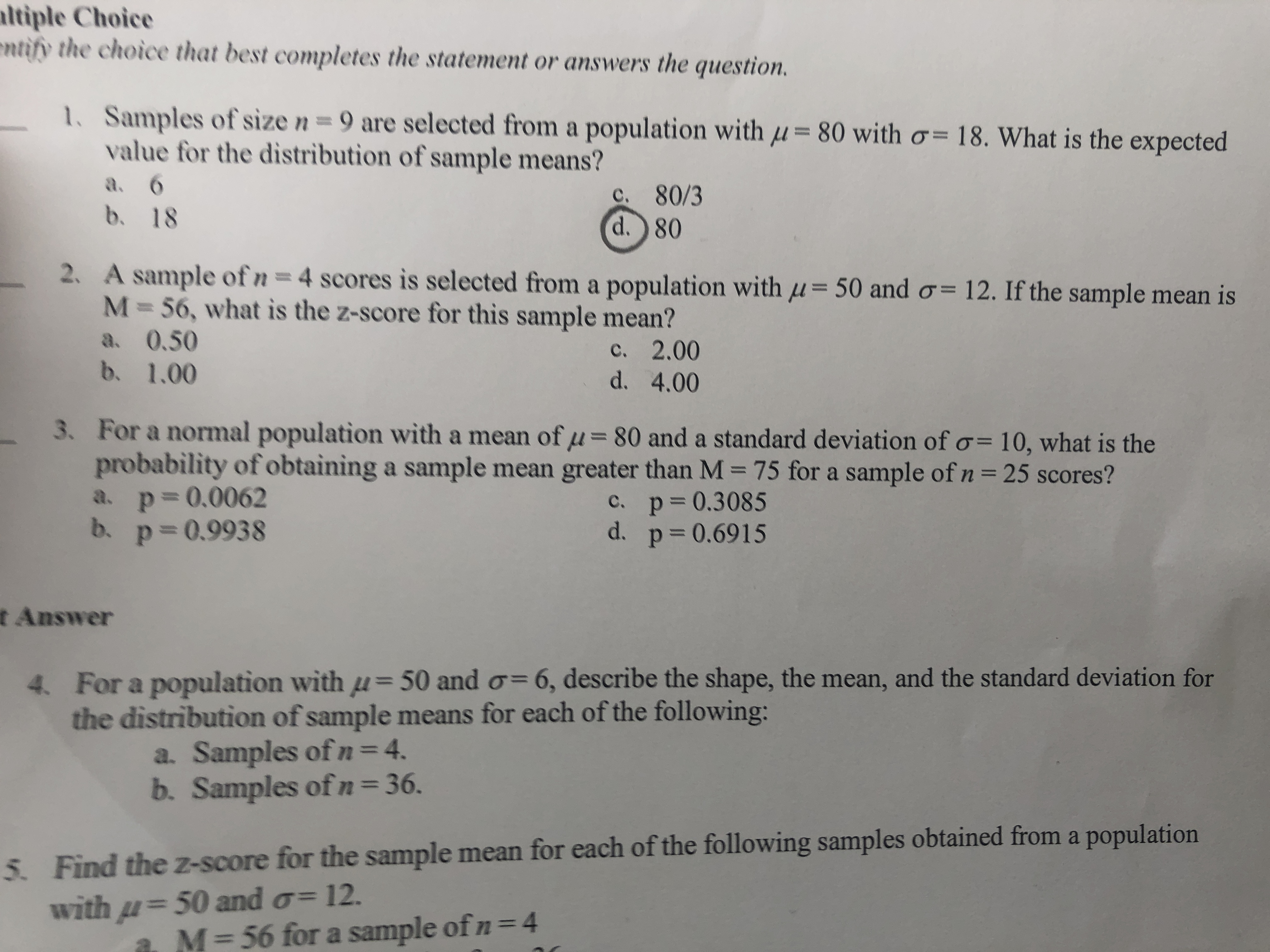# ltiple Choicentify the choice that best completes the statement or answers the question.1. Samples of size n 9 are selected from a population with = 80 with o= 18. What is the expectedvalue for the distribution of sample means?a. 6b.c. 80/3d.) 80182. A sample of n = 4 scores is selected froma population with uM=56, what is the z-score for this sample mean?50 and o= 12. If the sample mean isa. 0.50b. 1.00c. 2.00d. 4.003. For a normal population with a mean of u= 80 and a standard deviation of o- 10, what is theprobability of obtaining a sample mean greater than M 75 for a sample of n = 25 scores?a. p 0.0062b. p 0.993811C. p 0.3085d. p 0.69151t Answer6, describe the shape, the mean, and the standard deviation forFor a population with u= 50 and othe distribution of sample means for each of the following:a. Samples ofn = 4.b. Samples of n = 36.4.5. Find the z-score for the sample mean for each of the following samples obtained from a populationwith 50 and o 12.4M= 56 for a sample of n

Question

A sample of n=4 scores is selected from a population with m=50 and standard deviation of 12. If the sample mean is M=56, what is the z-score for this sample mean?help_outlineImage Transcriptioncloseltiple Choice ntify the choice that best completes the statement or answers the question. 1. Samples of size n 9 are selected from a population with = 80 with o= 18. What is the expected value for the distribution of sample means? a. 6 b. c. 80/3 d.) 80 18 2. A sample of n = 4 scores is selected froma population with u M=56, what is the z-score for this sample mean? 50 and o= 12. If the sample mean is a. 0.50 b. 1.00 c. 2.00 d. 4.00 3. For a normal population with a mean of u= 80 and a standard deviation of o- 10, what is the probability of obtaining a sample mean greater than M 75 for a sample of n = 25 scores? a. p 0.0062 b. p 0.9938 11 C. p 0.3085 d. p 0.6915 1 t Answer 6, describe the shape, the mean, and the standard deviation for For a population with u= 50 and o the distribution of sample means for each of the following: a. Samples ofn = 4. b. Samples of n = 36. 4. 5. Find the z-score for the sample mean for each of the following samples obtained from a population with 50 and o 12. 4 M= 56 for a sample of n fullscreen
check_circleExpert Solution
Step 1

It is given that the mean and standard dev...

### Want to see the full answer?

See Solution

#### Want to see this answer and more?

Solutions are written by subject experts who are available 24/7. Questions are typically answered within 1 hour*

See Solution
*Response times may vary by subject and question
Tagged in

### Statistics